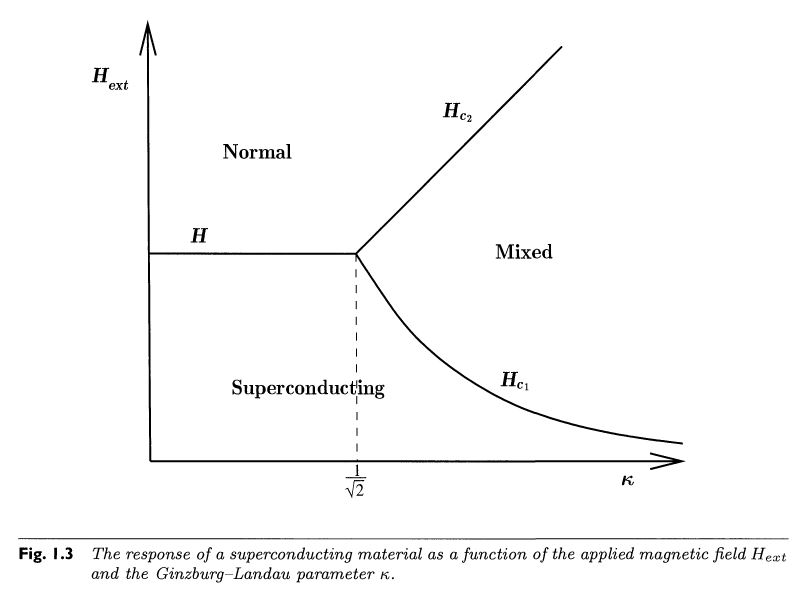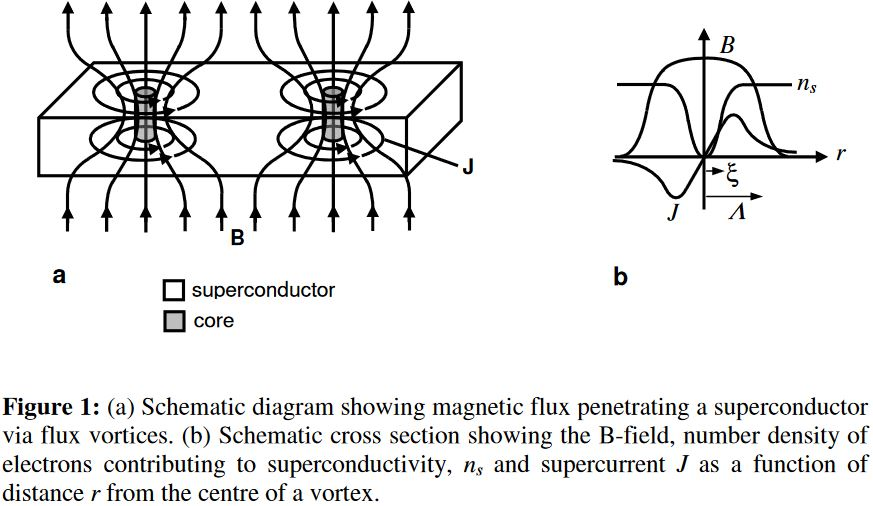Contents

# Contents

(…)

## Properties

### Magnetic flux quantization in type II superconductors

Due to the Meissner-Ochsenfeld effect, a superconductor placed in a sufficiently small external magnetic field $H_{ext} \lt H_{c_1}$ (aligned along some axis) “expels” that field , making the total magnetic field in the bulk of the superconductor vanish. However, as the ambient magnetic field exceeds a critical value $H_{c_1}$, this behaviour changes:

• for type I superconductors, the superconducting state simply breaks down as $H_{ext} \gt H_{c_1}$ and the ambient magnetic field fully penetrates the material as for any normal conductor;

• for type II superconductors the superconducting state eventually also breaks down as $H_{ext} \gt H_{c_2} \gt H_{c_1}$, but there is an intermediate parameter region $H_{c_1} \lt H_{ext} \lt H_{c_2}$ where both regimes mix:From Chapman 09

In this mixed regime, a finite number of elementary units of magnetic flux enter the superconductor, carried by little flux tubes inside vortices of electric currents: vortex strings (about a micron in diameter, e.g. Chapman 00, p. 559). Each vortex core carries one unit of magnetic flux – also called a fluxon – while at some small finite distance away from all vortices, the bulk magnetic flux in the superconductor still vanishes (mathematically: it vanishes at infinity):From Loudon-Midgley 09

At sufficiently large density these vortices form hexagonal patterns, first described by Abrikosov 57, whence also known as Abrikosov vortices.

This flux quantization in type II superconductors is traditionally explained via the effective field theory provided by the Landau-Ginzburg model; the derivation may be found reviewed in Chapman 00 (Section 2, culminating in (2.33)).

But, as indicated a little more explicitly in Alvarez-Gaumé 98 (Section IV.B, culminating below IV.11), the flux quantization as such is mathematically a direct consequence of the global topological nature of the electromagnetic field, the argument being the direct 2-dimensional analog of the quantization of instantons in QCD in 4d (see also at SU(2)-Instantons – From the correct maths to the traditional physics story) and in fact is but a slight variation of the argument for Dirac charge quantization of magnetic monopoles:

Namely, the electromagnetic field is a connection on a circle bundle, and hence the cohomology classifying space for the topological class of the electromagnetic field is the classifying space $B \mathrm{U}(1)$ of the circle group, which, being an Eilenberg-MacLane space $K(\mathbb{Z},2)$, has second homotopy group the integers:

$\pi_2(B \mathrm{U}(1)) \;\simeq\; \big\{ S^2 \to B \mathrm{U}(1)\big\}_{\big/hmpty} \;\simeq\; \mathbb{Z} \,.$

This implies that on every spacetime which looks, up to homotopy equivalence, like a 2-sphere to the electromagnetic field, the magnetic flux is identified with an element in the group of integers, hence is quantized in integral multiples of some unit flux.

For the case of a (hypothetical) magnetic monopole (e.g. a magnetically charged black hole), it is the spacetime around the monopole (the complement of its worldline $\mathbb{R}^{0,1}$ in the ambient (asymtptotically) Minkowski spacetime $\mathbb{R}^{3,1}$) which has the homotopy type of a 2-sphere $\mathbb{R}^{3,1} \setminus \mathbb{R}^{0,1} \,\simeq\, \mathbb{R}^{0,1} \times \mathbb{R}_{rad} \times S^2$; this implies the Dirac charge quantization of the magnetic monopole‘s magnetic charge:from SS21

For the case of the type II superconductor it is instead the transversal vanishing at infinity of the magnetic field (i.e. the Meissner-Ochsenfeld effect away from the vortices) which implies that the classifying map of the electromagnetic field sees not the full transversal Euclidean plane $\mathbb{R}^2$ but its one-point compactification $\big( \mathbb{R}^2 \big)^{cpt} \,\simeq\, S^2$, which introduces an effective 2-sphere topology onto spacetime (same as the 3-sphere in the discussion of Skyrmions and the 4-sphere in the discussion of instantons): $\mathbb{R}^{1,1} \times \big( \mathbb{R}^2 \big)^{cpt} \,\simeq\, \mathbb{R}^{1,1} \times S^2$. This implies the superconductor’s magnetic flux quantization:from SS21

The argument that is given in most references, via consideration of the period of the vector potential on a large circle around the superconductor (e.g. Timm 20, Section 5.3), is secretly just the analysis of this picture through the clutching construction (the direct 2d analog of the discussion at SU(2)-Instantons – From the correct maths to the traditional physics story).

### General

Review

• S. J. Chapman, A Hierarchy of Models for Type-II Superconductors, IAM Review Vol. 42, No. 4 (2000), pp. 555-598 (jstor:2653134)

• Carsten Timm, Theory of Superconductivity, 2020 (pdf)

See also:

Introducing Landau-Ginzburg models in superconductivity:

### Vortices and flux quantization

Original articles:

See also

First experimental detection of flux quantization in superconductors:

• Bascom S. Deaver, Jr., William M. Fairbank, Experimental Evidence for Quantized Flux in Superconducting Cylinders, Phys. Rev. Lett. 7, 43 – Published 15 (1961) (doi:10.1103/PhysRevLett.7.43)

• R. Doll, M. Näbauer, Experimental Proof of Magnetic Flux Quantization in a Superconducting Ring, Phys. Rev. Lett. 7, 51 (1961) (doi:10.1103/PhysRevLett.7.51)

More on the experimental detection magnetic flux quantization and vortices:

• J. C. Loudon, P. A. Midgley, Imaging Flux Vortices in Type II Superconductors with a Commercial Transmission Electron Microscope, Ultramicroscopy 109: 700-729, 2009 (arXiv:0807.2401)

More theoretically flavored discussion of the flux quantization mechanism:

On the vortex flux tubes as dynamical vortex strings:

### Anyons in topological superconductors

On anyon-excitations in topological superconductors.

Original proposal:

• Nicholas Read, Dmitry Green, Paired states of fermions in two dimensions with breaking of parity and time-reversal symmetries, and the fractional quantum Hall effect, Phys. Rev. B61:10267, 2000 (arXiv:cond-mat/9906453)

Review:

Further development:

• Meng Cheng, Victor Galitski, Sankar Das Sarma, Non-adiabatic Effects in the Braiding of Non-Abelian Anyons in Topological Superconductors, Phys. Rev. B 84, 104529 (2011) (arXiv:1106.2549)

via Majorana zero modes restricted to edges of topological insulators:

• Biao Lian, Xiao-Qi Sun, Abolhassan Vaezi, Xiao-Liang Qi, and Shou-Cheng Zhang, Topological quantum computation based on chiral Majorana fermions, PNAS October 23, 2018 115 (43) 10938-10942; first published October 8, 2018 (doi:10.1073/pnas.1810003115)

### Discussion via AdS/CFT

Discussion of superconductivity via AdS/CFT in condensed matter physics:

### High $T_c$-superconductivity

• M. C. Diamantini, C. A. Trugenberger, V. M. Vinokur, Topological Nature of High Temperature Superconductivity (arXiv:2009.01763)